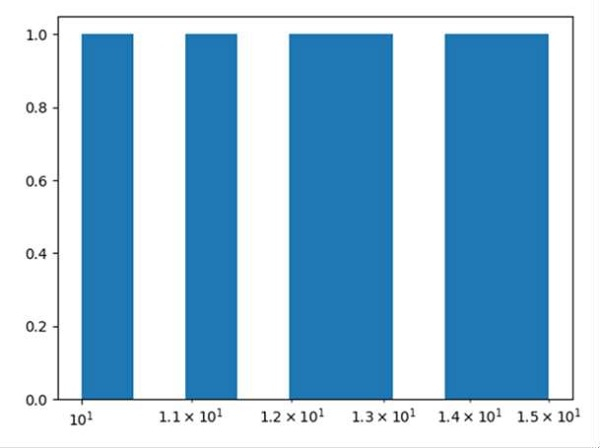# How to have logarithmic bins in a Python histogram?

We can set the logarithmic bins while plotting histogram using plt.hist(bin="").

## Steps

• Create an array x, where range is 100.

• Plot a histogram using plt.hist() method. We can pass logarithmic bins using logarithmic bins that returns numbers spaced evenly on a log scale.

• Get the current axes, creating one if necessary and set the X-axis scale.

• To show the figure, use plt.show() method.

## Example

from matplotlib import pyplot as plt
import numpy as np

x = np.array(range(100))

plt.hist(x, bins=np.logspace(start=np.log10(10), stop=np.log10(15), num=10))
plt.gca().set_xscale("log")
plt.show()

## OutputUpdated on: 16-Mar-2021

3K+ Views